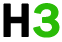C++基础 专题

C++ 变量作用域

• 在函数或一个代码块内部声明的变量，称为局部变量。
• 在函数参数的定义中声明的变量，称为形式参数。
• 在所有函数外部声明的变量，称为全局变量。

## 局部变量

```#include <iostream>
using namespace std;

int main ()
{
// 局部变量声明
int a, b;
int c;

// 实际初始化
a = 10;
b = 20;
c = a + b;

cout << c;     return 0;
} ```

## 全局变量

``````#include <iostream>
using namespace std;

// 全局变量声明
int g;

int main ()
{
// 局部变量声明
int a, b;

// 实际初始化
a = 10;
b = 20;
g = a + b;

cout << g;     return 0;
} ``````

``````#include <iostream>
using namespace std;

// 全局变量声明
int g = 20;

int main ()
{
// 局部变量声明
int g = 10;

cout << g;     return 0;
} ``````

```10
```

## 初始化局部变量和全局变量

int 0
char '\0'
float 0
double 0
pointer null